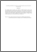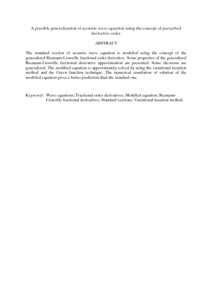# A possible generalization of acoustic wave equation using the concept of perturbed derivative order

## Citation

Atangana, Abdon and Kilicman, Adem (2013) A possible generalization of acoustic wave equation using the concept of perturbed derivative order. Mathematical Problems in Engineering, 2013. art. no. 696597. pp. 1-6. ISSN 1024-123X

## Abstract

The standard version of acoustic wave equation is modified using the concept of the generalized Riemann-Liouville fractional order derivative. Some properties of the generalized Riemann-Liouville fractional derivative approximation are presented. Some theorems are generalized. The modified equation is approximately solved by using the variational iteration method and the Green function technique. The numerical simulation of solution of the modified equation gives a better prediction than the standard one.Preview
PDF (Abstract)
A possible generalization of acoustic wave equation using the concept of perturbed derivative order.pdfView Item